# Faces Edges and Vertices – Properties of 3D Shapes – Maths

3D Shapes – Faces, Edges and Vertices

• Cuboid
• Cube
• Cone
• Sphere
• Square Pyramid
• Triangular Prism
• Triangular Pyramid

## 3D Shape – Faces, Edges and Vertices

Face is a flat surface that forms part of the boundary of a solid object.
A vertex is a corner.
An edge is a line segment joining two vertex.

### Faces, Edges and Vertices – Cuboid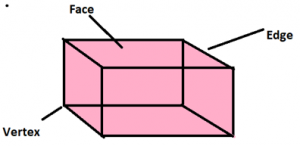A cuboid has six rectangular faces.

A cuboid has 8 vertices.

A cuboid has 12 edges.

### Cube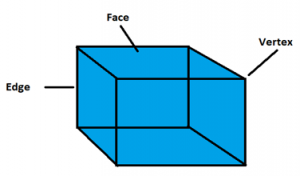A cube has six square faces.

A cube has eight vertices.

A cube has 12 edges.

### Cone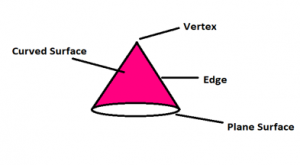A cone has one plane surface (i.e base) and one curved lateral surface.

A cone has 1 vertex.

A cone has 1 circular edge.

### Faces, Edges and Vertices – Cylinder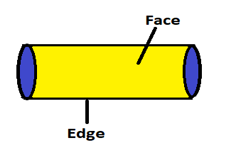A cylinder has a curved lateral surface and two circular faces at its ends.

A cylinder has no corner or vertex.

A cylinder has 2 circular edges.

### Sphere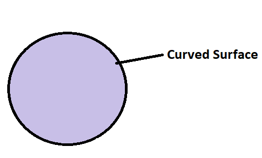A sphere has a curved surface.

A sphere has no vertex.

A sphere has no edges.

### Square Pyramid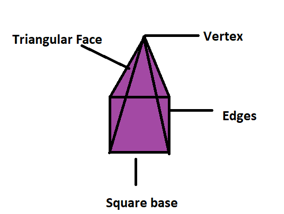A square pyramid has 4 lateral triangular faces and 1 square base.

A square pyramid has 5 vertices.

A square pyramid 8 edges.

### Triangular Prism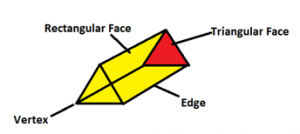A triangular prism has 3 rectangular lateral faces and 2 triangular bases.

A triangular prism has 6 vertices.

A triangular prism has 9 edges.

### Faces, Edges and Vertices – Triangular Pyramid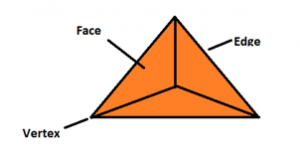A triangular pyramid has 3 triangular lateral faces, 1 triangular base.

A triangular pyramid has 4 vertices.

A triangular pyramid has  6 edges.

### 2 thoughts on “Faces Edges and Vertices – Properties of 3D Shapes – Maths”

1.wow

Reply
2.Thanks for the good content…..

Reply